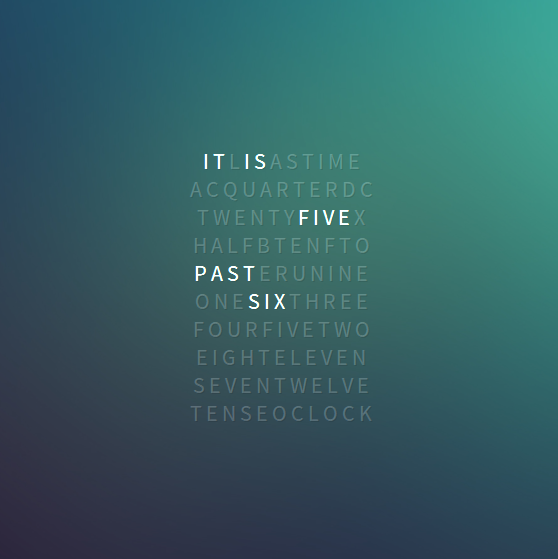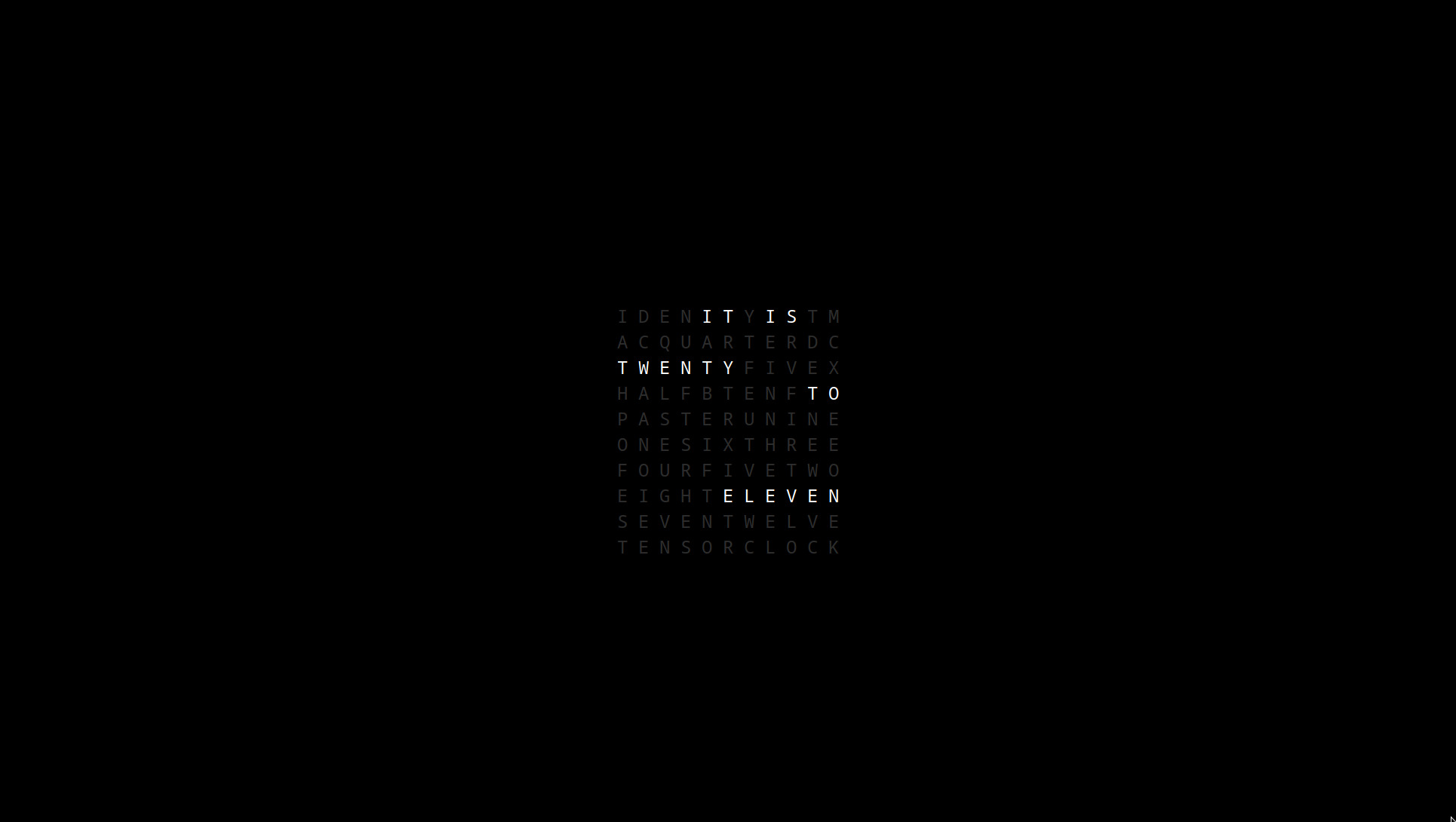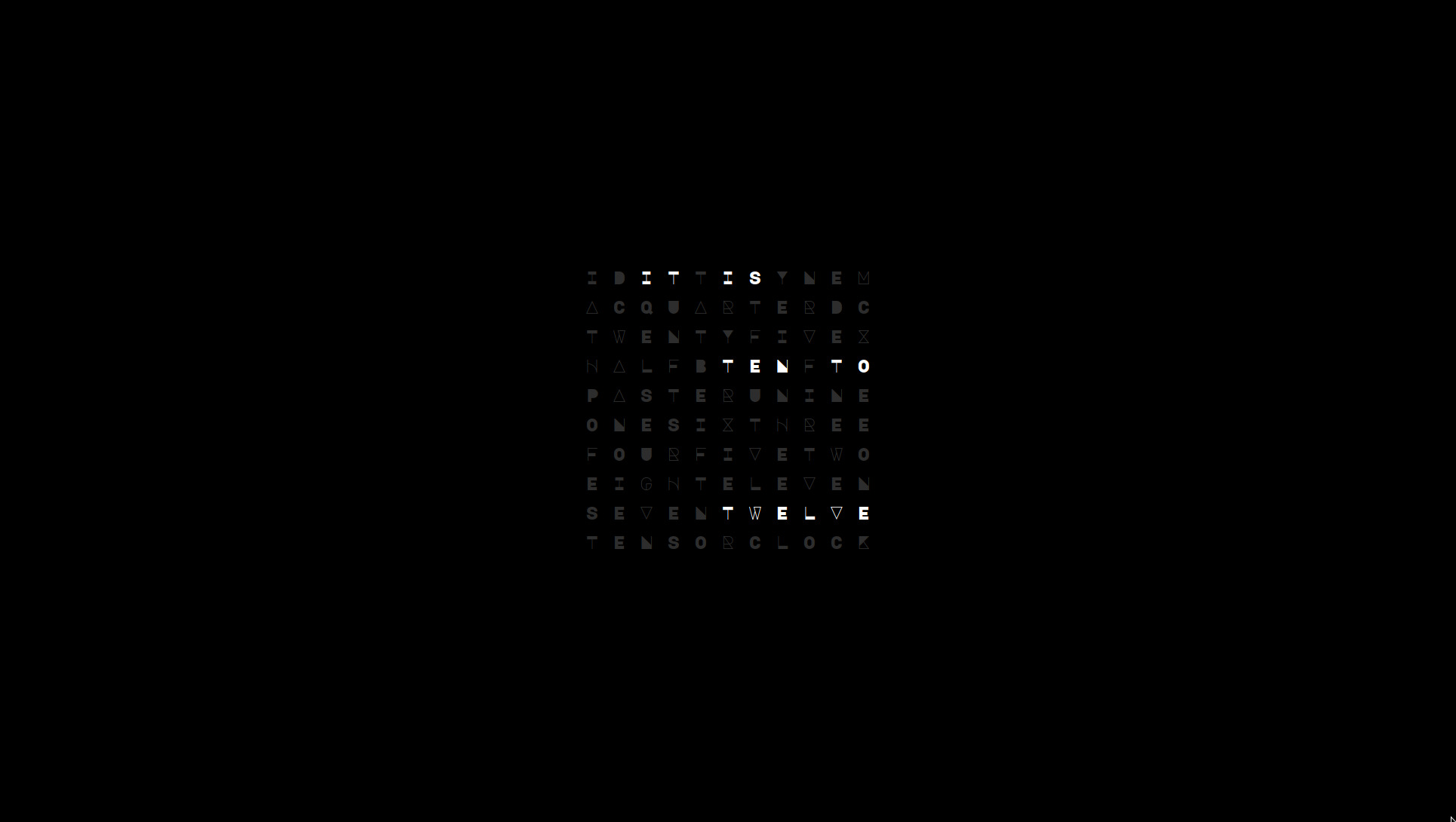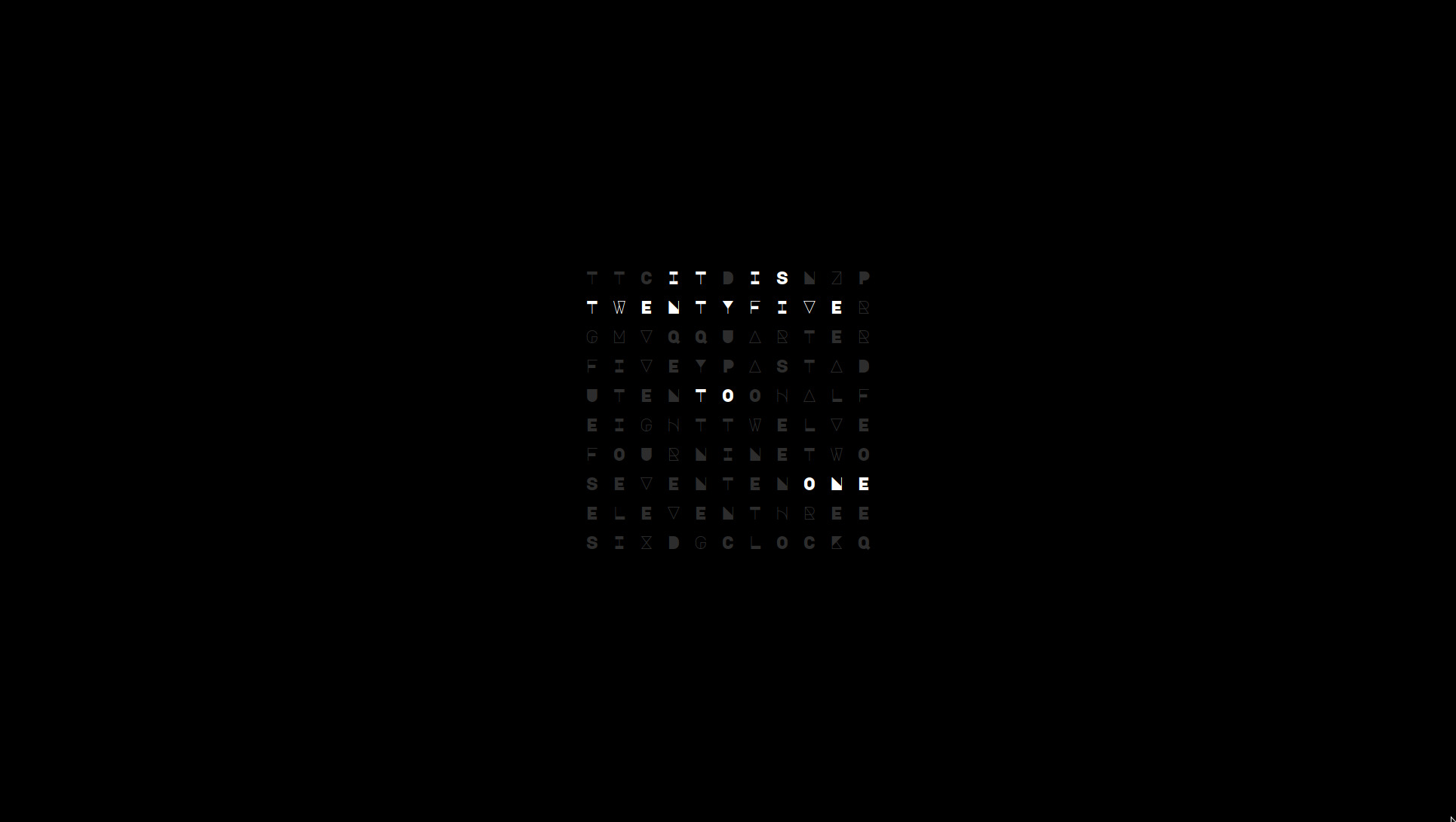# conky qclocktwo

friday, 26 july 2019

the statement design of QClockTwo was popularized on Linux desktops with mowgli-writes conky port.i adapted this as a screensaver with an opaque black background (and the much better looking Noto Sans Mono font which produces a squared presentation), centering it on my screen.

## where it is

it didn’t take long before i altered the conky code to shift the position of the top “IT IS” line with each 5 minute refresh to avoid display burn in, the conky syntax of which is very dense (and lacking readability)..

`conky.text = [[` `...` `\${if_match \${exec date +"%M"} < 5}\${alignc}\${color}I T \${color1}E \${color}I S \${color1}I D Y N T M\${endif}\${if_match \${exec date +"%M"} >= 5}\${if_match \${exec date +"%M"} < 10}\${color1}I \${color}I T \${color1}N \${color}I S \${color1}D Y E T M\${endif}\${endif}\${if_match \${exec date +"%M"} >= 10}\${if_match \${exec date +"%M"} < 15}\${color1}I D \${color}I T \${color1}T \${color}I S \${color1}Y N E M\${endif}\${endif}\${if_match \${exec date +"%M"} >= 15}\${if_match \${exec date +"%M"} < 20}\${color1}I D E \${color}I T \${color1}N \${color}I S \${color1}T Y M\${endif}\${endif}\${if_match \${exec date +"%M"} >= 20}\${if_match \${exec date +"%M"} < 25}\${color1}I D E N \${color}I T \${color1}Y \${color}I S \${color1}T M\${endif}\${endif}\${if_match \${exec date +"%M"} >= 25}\${if_match \${exec date +"%M"} < 30}\${color1}I D E N T \${color}I T \${color1}Y \${color}I S \${color1}M\${endif}\${endif}\${if_match \${exec date +"%M"} >= 30}\${if_match \${exec date +"%M"} < 35}\${color1}I D E N T Y \${color}I T \${color1}M \${color}I S\${color1}\${endif}\${endif}\${if_match \${exec date +"%M"} >= 35}\${if_match \${exec date +"%M"} < 40}\${color1}I D E N T \${color}I T \${color1}Y \${color}I S \${color1}M\${endif}\${endif}\${if_match \${exec date +"%M"} >= 40}\${if_match \${exec date +"%M"} < 45}\${color1}I D E N \${color}I T \${color1}Y \${color}I S \${color1}T M\${endif}\${if_match \${exec date +"%M"} >= 45}\${if_match \${exec date +"%M"} < 50}\${color1}I D E \${color}I T \${color1}N \${color}I S \${color1}T Y M\${endif}\${endif}\${if_match \${exec date +"%M"} >= 50}\${if_match \${exec date +"%M"} < 55}\${color1}I D \${color}I T \${color1}T \${color}I S \${color1}Y N E M\${endif}\${endif}\${if_match \${exec date +"%M"} >= 55}\${color1}I \${color}I T \${color1}N \${color}I S \${color1}D Y E T M\${endif}\${endif}`

This statement alone begs for a different approach!## major mono display

discovering the major</span> mono display Google font added a more interesting flair to the clock..A conky constant voffset is required with each line of the display to garner a squared presentation.

## urandom and shuf

by spawning an external process to generate the conky text instead of using conky script or lua language..

`conky.text = [` `...` `\${execp conky qclocktwo '`

`<span class="center">✱&nbsp;&nbsp;✱&nbsp;&nbsp;✱</span>'}`

`](http://thedarnedestthing.com/` `...` `\${execp conky qclocktwo '`

`<span class="center">✱&nbsp;&nbsp;✱&nbsp;&nbsp;✱</span>'}`

`);`

*nix commands urandom and shuf can be used in shell functions to further randomize the content placement of the clock time in a shell script (further reducing burn in but primarily for visual permutations—clocks across multiple computers will all display the same time with line variations)..

`#!/usr/bin/dash` `...` `Q='{color}'` `_='{color1}'` `esc='s/[{]/\$&/g'` ` ` `ch() { cat /dev/urandom | tr -dc 'A-Z' | head -c \$1 | sed 's/./& /g'; }` `xs() { echo "\${_}\$(ch 1)\${Q}"; }` `nx() { for j in \$(seq 1 \$1) ;do echo "\n\${_}\$(ch 1)"; done; }` `shuffle() { echo "\$@" | shuf | tr -d '\n'; }` `draw() { [ "\$qclock" ] && echo "\$voffset\$offset\$qclock" | sed "s/ \$//; \$esc" >\$QCLOCK:\$1; }` `...`Refer to the dotfiles for script details of the content parsing rules (which are much easier to write and maintain compared to conky script).

Sometimes.. one has too much time on their hand!